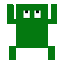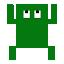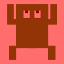# Using command line tools for image comparisons

## Introduction

Squish supports several image comparison methods: pixel strict, pixel fuzzy, histogram and correlation comparison. All of them are used in so called image verification points (Screenshot Verification Point dialog). You can create these verifications during test recording and later on modify them in the Squish IDE.

For lots of cases that is usually enough but sometimes you might need a bit more. For example, when you would like to check some images which are dynamically created or maybe when you don't have the images in advance. In that case you can use the following command line tools: simplecompare, nchcompare and correlationcompare. You can find these tools in the folder 'bin' of each Squish installation.

## Example

With a little bit of scripting you can easily use (call) these tools in your test scripts as well:

`import os`
`import subprocess`

`def simplecompare(image1, image2, threshold=0, tolerance=0):`
`    args = [os.path.join(os.environ["SQUISH_PREFIX"], "bin",`
`"simplecompare"),`` image1, image2, str(threshold), str(tolerance)]`
`    ``return subprocess.call(args) == 0`

`def nchcompare(image1, image2, bincount=4, threshold=0):`
`    ``args = [os.path.join(os.environ["SQUISH_PREFIX"], "bin", "nchcompare"),        `i`mage1, image2, str(bincount), str(threshold)]`
`    ``return subprocess.call(args) == 0`

`def correlationcompare(image1, image2, threshold=90):`
`    ``args = [os.path.join(os.environ["SQUISH_PREFIX"], "bin",``         "correlationcompare"),` `image1, image2, str(threshold)]`
`    ``return subprocess.call(args) == 0`

The first function calls the tool simplecompare which accepts four arguments. First two arguments are images for comparison, the third is the maximal allowed number of different pixels (in percents) and the fourth is the tolerance i.e. how much two pixels can differ from each other so that they can still be considered to be the same.

The second function calls the tool nchcompare which calculates the color histogram of the specified images and if the histograms differ more than the threshold, the function returns false.

Similarly the third function calls the tool correlationcompare which calculates a correlation between two images and returns false if the correlation is below the specified threshold.

## Usage

You can take a screenshot i.e. "grab" the picture of the widgets which you would like to test using the function grabWidget. That function returns an Image object which you can save to some temporary location and then pass its path to the functions above.

You can also build some other functions on top of the script functions above. For example you can make a function which checks whether a difference between histograms of two pictures is within a certain range:

`def histogramDiff(image1, image2, bincount, minthreshold, maxthreshold):`
`    return nchcompare(image1, image2, bincount, maxthreshold) \`
`    and not nchcompare(image1, image2, bincount, minthreshold)`

That can be used to check whether, for example, a red color overlay is displayed over an image:`def checkHistograms():`
`    nchTemp = os.path.join(squishinfo.resultDir, "nchTmp")`
`    os.mkdir(nchTemp) # folder for temporary images`
`    image1File = os.path.join(nchTemp, "image1.png")`
`    image2File = os.path.join(nchTemp, "image2.png")`

`    imgage1 = grabWidget(waitForObject(":Image1"))`
`    imgage2 = grabWidget(waitForObject(":Image2"))`
`    imgage1.save(image1File, "PNG")`
`    imgage2.save(image2File, "PNG")`
`    test.verify(histogramDiff(image1File, image2File, 4, 2.5, 3.5))`

The Qt Company acquired froglogic GmbH in order to bring the functionality of their market-leading automated testing suite of tools to our comprehensive quality assurance offering.